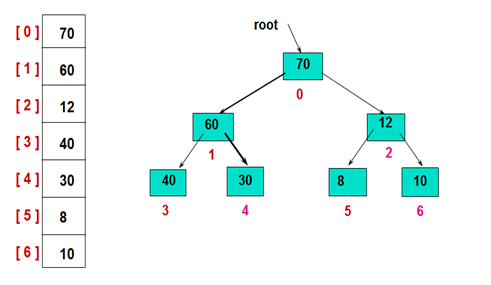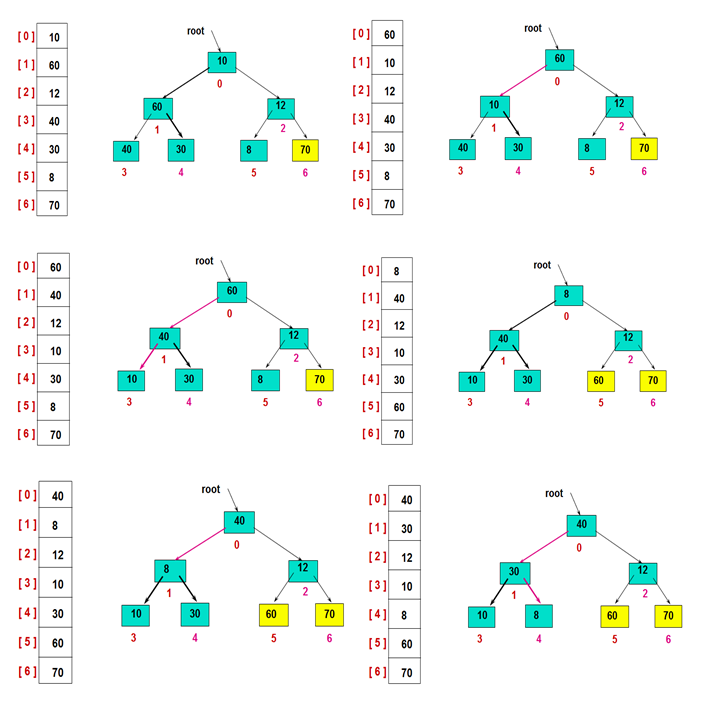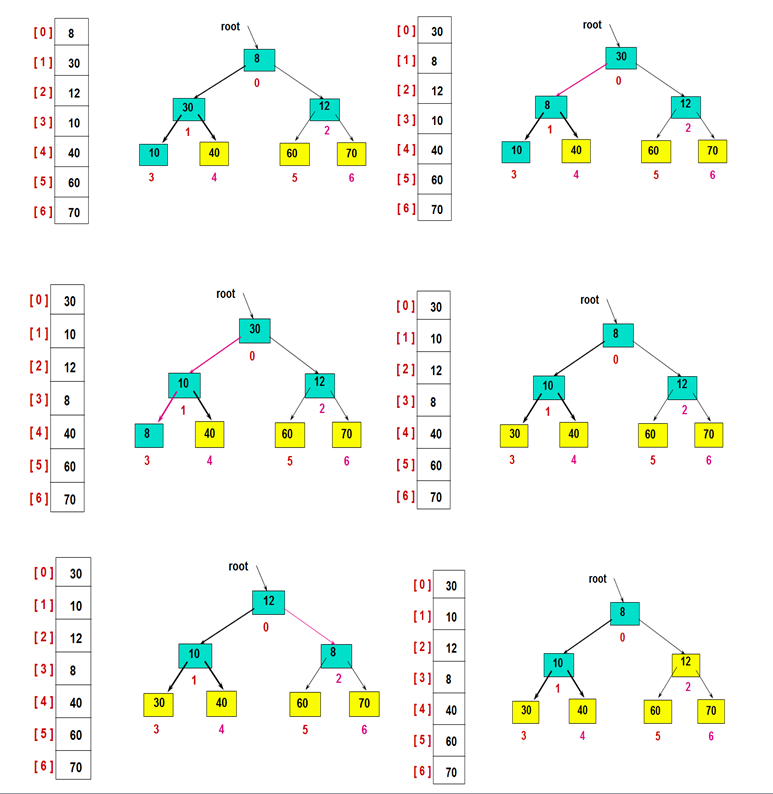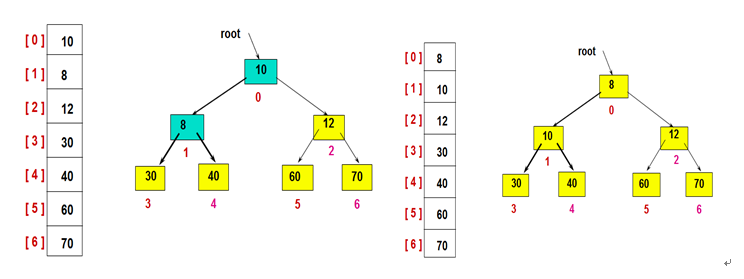# 【java面试】算法篇之堆排序

1.将数组构造成初始堆（若想升序则建立大根堆，若想降序，则建立小根堆）2.堆排序处理public void HeapAdjust(int[] array, int parent, int length) {

int temp = array[parent]; // temp保存当前父节点

int child = 2 * parent + 1; // 先获得左孩子

while (child < length) {

// 如果有右孩子结点，并且右孩子结点的值大于左孩子结点，则选取右孩子结点

if (child + 1 < length && array[child] < array[child + 1]) {

child++;

}

// 如果父结点的值已经大于孩子结点的值，则直接结束

if (temp >= array[child])

break;

// 把孩子结点的值赋给父结点

array[parent] = array[child];

// 选取孩子结点的左孩子结点,继续向下筛选

parent = child;

child = 2 * child + 1;

}

array[parent] = temp;

}

public void heapSort(int[] list) {

// 循环建立初始堆

for (int i = list.length / 2; i >= 0; i--) {

HeapAdjust(list, i, list.length - 1);

}

// 进行n-1次循环，完成排序

for (int i = list.length - 1; i > 0; i--) {

// 最后一个元素和第一元素进行交换

int temp = list[i];

list[i] = list;

list = temp;

// 筛选 R 结点，得到i-1个结点的堆

System.out.format("第 %d 趟: \t", list.length - i);

printPart(list, 0, list.length - 1);

}

}

## 堆排序算法的总体情况

 排序类别 排序方法 时间复杂度 空间复杂度 稳定性 复杂性 平均情况 最坏情况 最好情况 选择排序 堆排序 O(nlog2n) O(nlog2n) O(nlog2n) O(1) 不稳定 较复杂